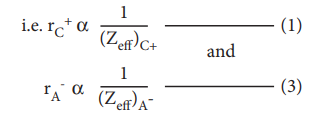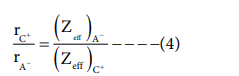Home | | Chemistry 11th std | Ionic radius

## Chapter: 11th Chemistry : UNIT 3 : Periodic Classification of Elements

It is defined as the distance from the centre of the nucleus of the ion up to which it exerts its influence on the electron cloud of the ion.

It is defined as the distance from the centre of the nucleus of the ion up to which it exerts its influence on the electron cloud of the ion. Ionic radius of uni-univalent crystal can be calculated using Pauling's method from the inter ionic distance between the nuclei of the cation and anion. Pauling assumed that ions present in a crystal lattice are perfect spheres, and they are in contact with each other therefore,

d = rC+ + rA- ------------------                                                                                            (1)

Where d is the distance between the centre of the nucleus of cation C+ and anion A- and rC+, r A- are the radius of the cation and anion respectively.

Pauling also assumed that the radius of the ion having noble gas electronic configuration (Na+ and Cl-having 1s2 2s2, 2p6 configuration) is inversely proportional to the effective nuclear charge felt at the periphery of the ion.Where Zeff is the effective nuclear charge and Zeff = Z - S

Dividing the equation 1 by 3On solving equation (1) and ( 4 ) the values of rC+and rA- can be obtained

Let us explain this method by calculating the ionic radii of Na+ and F- in NaF crystal whose interionic distance is equal to 231 pm .Study Material, Lecturing Notes, Assignment, Reference, Wiki description explanation, brief detail
11th Chemistry : UNIT 3 : Periodic Classification of Elements : Ionic radius |